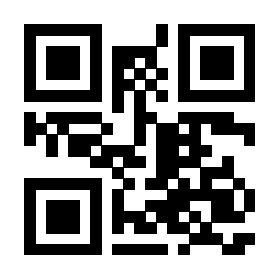# 环境中修改回车符的行为

\begin{choices}
\item 选
\item 项
\end{choices}

\begin{choices}

\end{choices}

\def\everyEnter#1\n{\doSomethingWith{#1}%分离出一行的内容进行处理
\@ifnextchar\end{\relax}{\everyEnter}}%如果回车后是 \end 就休息，否则重复 \everyEnter

\newenvironment{makeEnterMacro}{\catcode\^^M\active \everyEnter}
{\catcode\^^M=10}

MWE 如下

\documentclass[UTF8]{ctexart}\pagestyle{empty}
\begin{document}
\section{改变回车行为示例}

\catcode\^^M\active
\let^^M\TeX\relax

\def^^M{我是回车\par}

\catcode\^^M=10 % 恢复正常回车

\section{在环境中 手工 改变回车行为}

\begin{center}
\catcode\^^M\active \let^^M\LaTeX\relax

\end{center}

\section{问题来了：定义一个改变回车的环境，怎么就报错了呢}

\newenvironment{makeEnterMacro}{\catcode\^^M\active \let^^M\LaTeX\relax}{\catcode\^^M=10}

% newenvironment 有问题，以下瞎改
%\def\cc{\protect\catcode\^^M=13\relax\def\zz##1^^M{【##1】}\zz}
%\def\endcc{\catcode\^^M=10}

%\begin{makeEnterMacro}

%\end{makeEnterMacro}

\end{document}

（或者说，在 LaTeX 中实现一种简约的列表数据结构）

0

617

TeX 从文件中读入一行，去掉行终止符（catcode=5），去掉行尾空格，插入 \endlinechar，一般为 return 字符（ASCII 13）。

\catcode\^^M=13 % 这里最好使用注释符
\newenvironment{makeEnterMacro}{\catcode\^^M\active \let^^M\LaTeX\relax}{\catcode\^^M=5 }% 同上
\catcode\^^M=5 % 同上

MWE：

\documentclass[UTF8]{ctexart}\pagestyle{empty}
\begin{document}

\catcode\^^M=13 %
\newenvironment{makeEnterMacro}{\catcode\^^M\active \let^^M\LaTeX\relax}{\catcode\^^M=5 }%
\catcode\^^M=5 %

\begin{makeEnterMacro}

\end{makeEnterMacro}

\end{document}\makeatletter
\newenvironment{mychoices}
{\def\obeyedline{\@ifnextchar\end{\par}{\item}}\obeylines
\begin{itemize}}
{\end{itemize}}
\makeatother

\begin{mychoices}

\end{mychoices}`
1 个回答

### 撰写答案